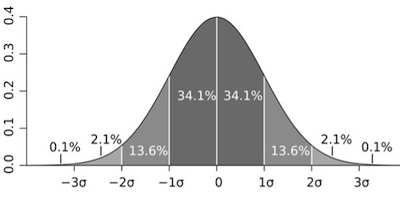# Descriptive Statistics

## Objective

Calculate population percentages using the standard deviation.

## Common Core Standards

### Core Standards

?

• HSS-ID.A.4 — Use the mean and standard deviation of a data set to fit it to a normal distribution and to estimate population percentages. Recognize that there are data sets for which such a procedure is not appropriate. Use calculators, spreadsheets, and tables to estimate areas under the normal curve.

?

• 7.SP.B.3

• 7.SP.B.4

## Criteria for Success

?

1. Identify that the number of standard deviations (both positive and negative) from the mean are represented in a normal distribution.
2. Derive the percentage of data within one, two, and three standard deviations of the mean.
3. Use the normal distribution as a guide to determine the percentage of data in a contextual situation, given the mean and standard deviation.
4. Interpret a contextual situation and make comparisons based on the population percentages of a normal distribution.

## Anchor Problems

?

### Problem 1### Problem 2

At a certain pizza delivery place, the average delivery time is 42 minutes with a standard deviation of 3 minutes.
Based on this information, would you purchase a pizza from this company? Why or why not?

### Problem 3

A particular college has all students take a placement test. If you score in the top 16% of those who have been admitted, you are offered a scholarship.

The range of scores you can get on the placement test are 100 to 800. The scores for the cohort are normally distributed, and the average is 550. The standard deviation for the administration of this test to the cohort was 75 points.

You get a 650 on the test. Do you get offered the scholarship?

## Problem Set

?

The following resources include problems and activities aligned to the objective of the lesson that can be used to create your own problem set.

Include problems where you ask students to interpret interquartile range as well as standard deviation in context.